# Nickzom Calculator Solves Molar Concentration in Chemistry

According to Wikipedia,

Molar concentration (also called molarityamount concentration or substance concentration) is a measure of the concentration of a chemical species, in particular of a solute in a solution, in terms of amount of substance per unit volume of solution. In chemistry, the most commonly used unit for molarity is the number of moles per litre, having the unit symbol mol/L. A solution with a concentration of 1 mol/L is said to be 1 molar, commonly designated as 1 M.

Nickzom Calculator+ (Professional Version) – The Calculator Encyclopedia requires two parameters to get the answer for a molar concentration in Chemistry such as:

• Number of Moles
• Volume

One can access Nickzom Calculator+ (Professional Version) – The Calculator Encyclopedia via any of these channels:

First, go to the calculator map, scroll down to the Chemistry section and click on Basic Chemistry.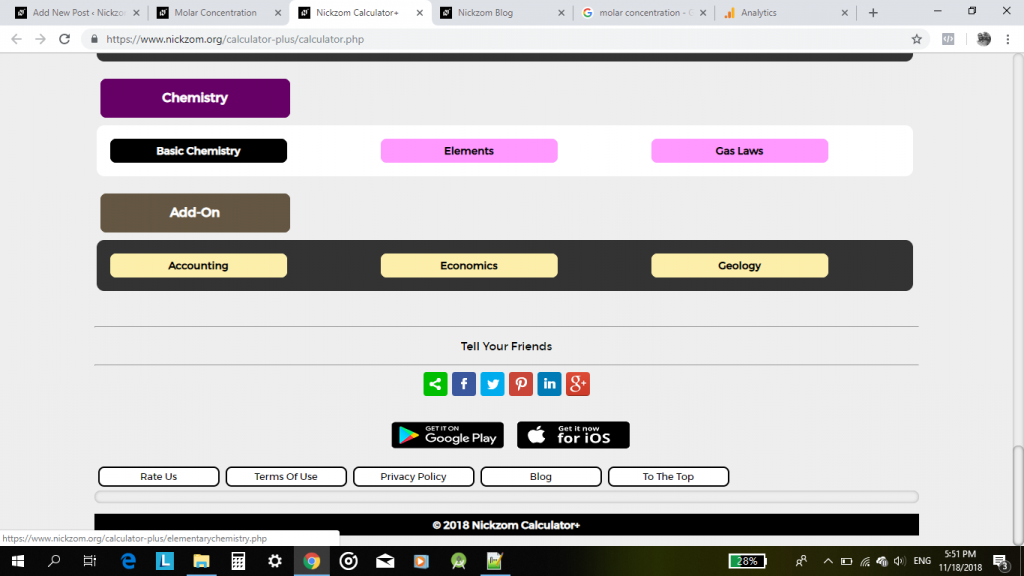Now, click on Molar Concentration in Chemistry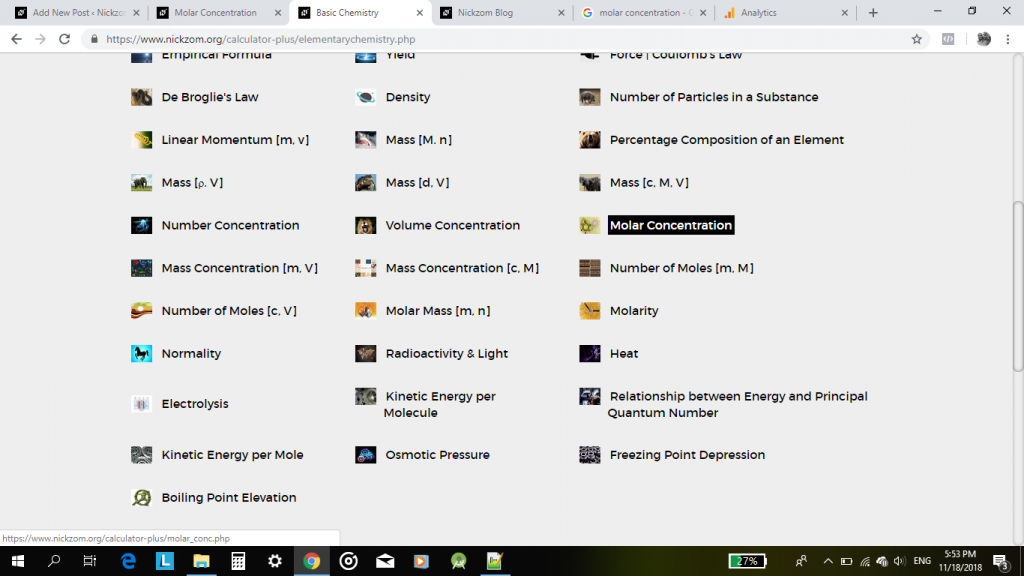It would then display the input page for you to enter the values of the required parameters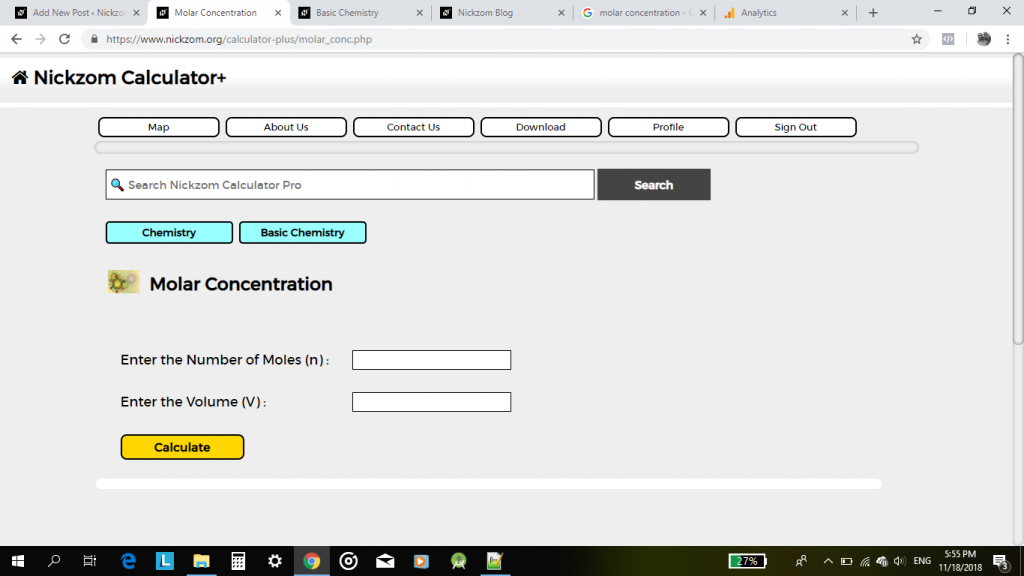Now, enter the values of the number of moles and volume.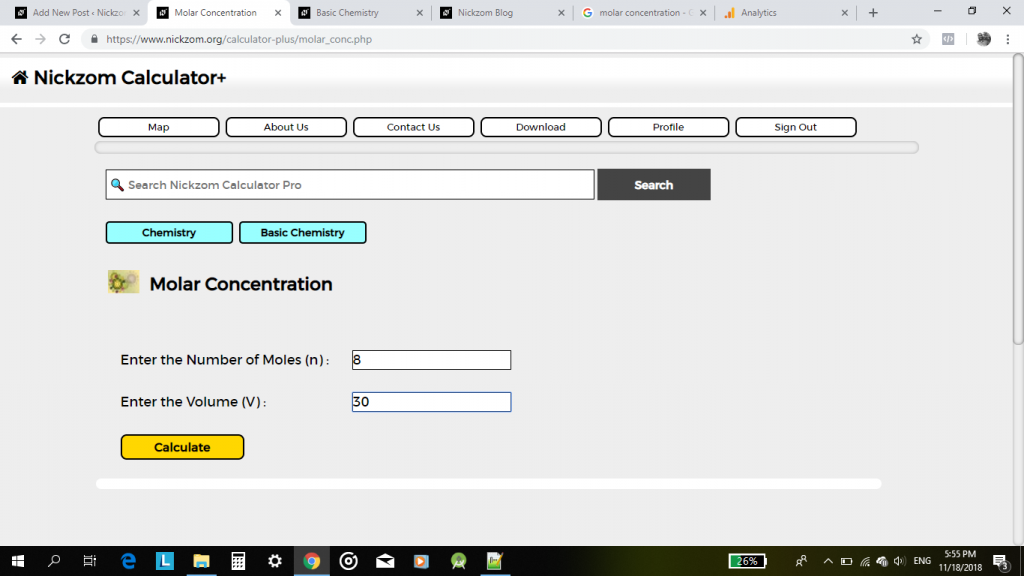Finally, click on Calculate to display the result.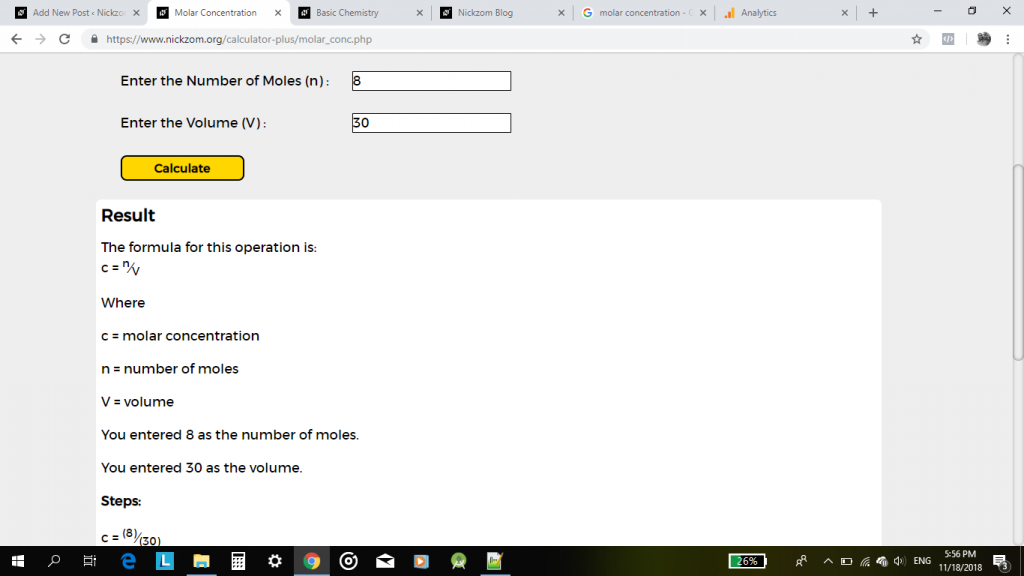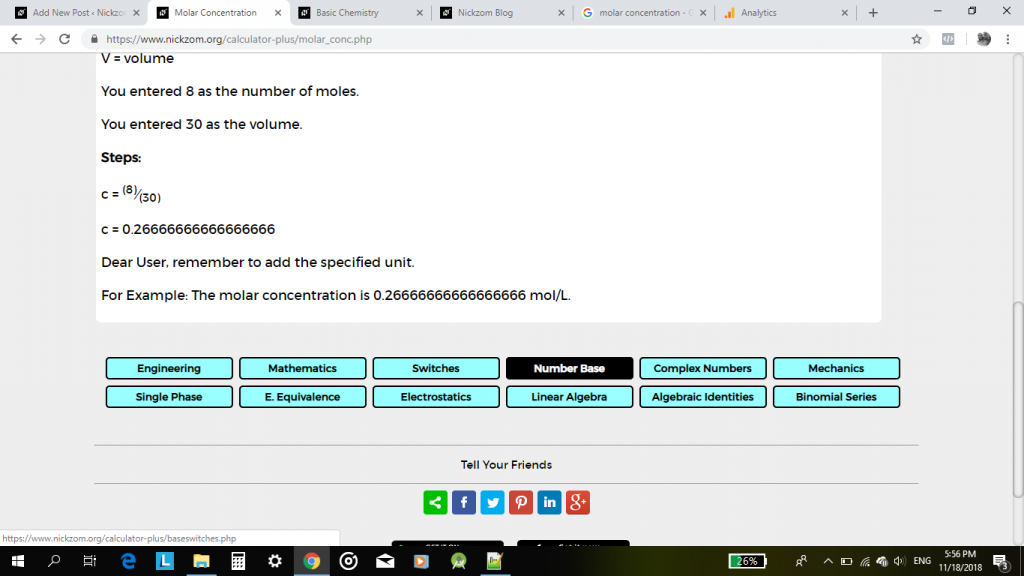Is it not wonderful? Thank you for reading our post on Nickzom Blog.